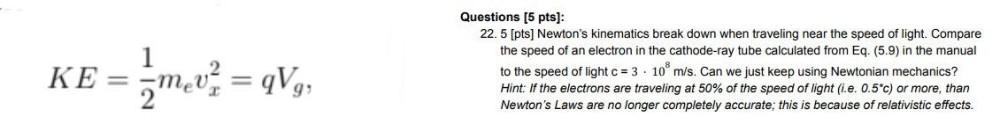Question:

# 1 KER, Questions (5 pts]: 22.5 [pts] Newton's kinematics break down when traveling near the speed of light. Compare the speed of1 KER, Questions (5 pts]: 22.5 [pts] Newton's kinematics break down when traveling near the speed of light. Compare the speed of an electron in the cathode-ray tube calculated from Eq. (5.9) in the manual to the speed of light c = 3 . 10 m/s. Can we just keep using Newtonian mechanics? Hint: If the electrons are traveling at 50% of the speed of light (i.e. 0.5*c) or more than Newton's Laws are no longer completely accurate; this is because of relativistic effects. 2